# Working Of Keras Functional API

Keras  Python library is a multi-backend and multi-platform that is more flexible ways of creating and working on complex deep learning models fast and easy. It minimizes the number of steps used in common use cases, and it provides clear and actionable feedback upon user error.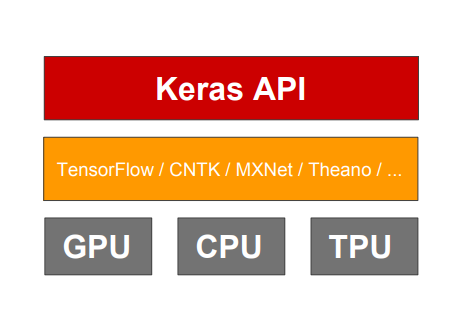Module: tensorflow.keras (tf.keras)

Tensor computations are not defined by the Keras and are performed at the lower levels i.e. Tensorflow, Theano, and CNTK. In a nutshell, it can be said that Keras is a reference implementation that may be considered as wrapper over TensorFlow. TensorFlow 2.0 adopted Keras API specification as to their default, high-level API for building and training deep learning models. TensorFlow comes with another, self-contained implementation of the Keras API specification:

tf.keras

and exist in the TensorFlow package itself and the user does not have to install the Keras package explicitly.

Sequential and Functional API's

Keras sequential API helps to create sequential models layer by layer, however, it does not allow the developer to create models with shareable layers or layers with multiple input and output.  These limitations of sequential API are overcome by the Keras functional API which can handle non-linear models with shared layers and multiple inputs and outputs.

 SEQUENTIAL API FUNCTIONAL API Beginner-friendly Yes Modeling Capability Directed path graph Directed acyclic graph Allows multi-input,multi-output models Yes

Pseudocode:

"

import keras

from keras import layers

inputs= keras.Input(shape=(10,))

x=layers.Dense(20,activation='relu')(x)

x=layers.Dense(20,activation='relu')(x)

outputs = layers.Dense(10,activation='softmax')(x)

model=keras.Model(inputs,outputs)

model.fit(x,y,epochs=10,batch_size=32)

"

With functional API we can also use a model with the same architecture and weights dynamically as we use layers by calling it on tensors, that is, in functional API the models are also callable.

Here's a good use case for the active API: models with lots of inputs and outputs. An effective API makes it easy to trick a large number of deep models into one.

Let us consider the following model. We want to predict how many retweets and likes the news headlines to get on Twitter. The main input to the model will be the title itself, as is the word sequence, but to spice things up, our model will also have helpful input, receiving additional data such as the time of day when the header was added, etc. The model will also be employed with two loss functions. Using a great deal of initial loss function in the model is a great way to do deeper models. We will have a model as: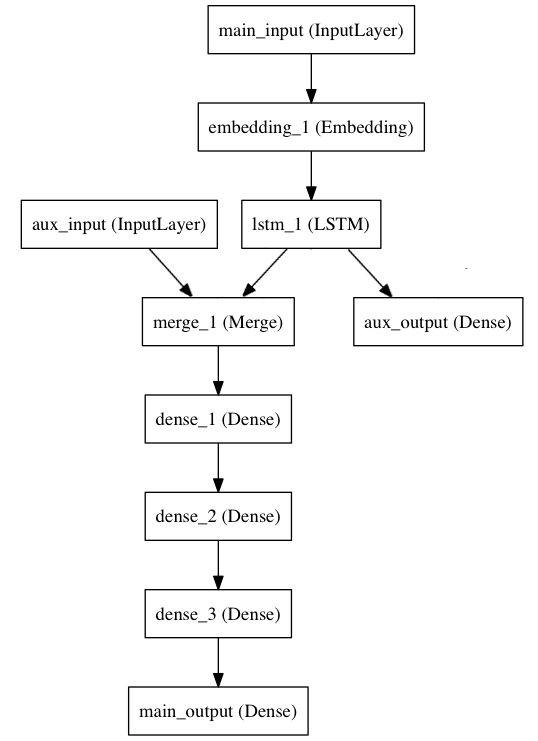Keras Objects-

Keras has three main objects:-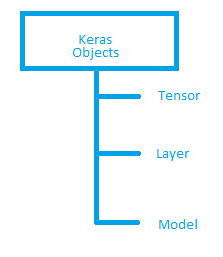Other than these we have objects such as NetworkInitializerOptimizer,and Callback

Keras Tensor: It is produced by an input function. A tensor is a generalization of vectors and matrices to potentially higher dimensions. That one was clear from the beginning. For example,

from keras.layers import Input

x = Input(batch_shape=(10000, 100))

Usually, a tensor is an object from the underlying backends i.e. Theano , TensorFlow , MXnet, PaidML or CNTK, that we augment with certain attributes that allow us to build a Keras model just by knowing the inputs and outputs of the model.

Layer: A Layer defines a transformation. Layer accepts Keras tensor(s) as input, transforms the input(s), and outputs Keras tensor(s). Layers can do a wide variety of transformations. DenseActivationReshapeConv2D, and LSTM are all Layers derived from the abstract layer class.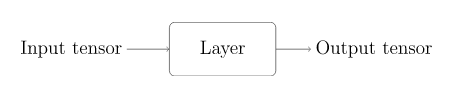Usage:

from keras.layers import Dense

dense_layer = Dense(units=10, activation='relu')

dense_layer = Dense(units=10, activation='softmax')

type(dense_layer)

Output: keras.layers.core.Dense

Keras Graph:

When we use a backend (such as TensorFlow), we create a graph that describes the combinations you intend to perform. This graph can be optimized when using a passion that is actively or explicitly used (such as by sess.run () on TensorFlow).  Even though Keras hides a lot of low-level backend complexity, Keras computation is still based on a graph. Understanding this Keras graph is important to fully understand the Functional API. In fact, by using the Functional API you are specifying a Keras graph. Typically, Keras graph is represented much more compactly than a backend graph. The pictures below show the Keras graph and the corresponding TensorFlow graph for the same network.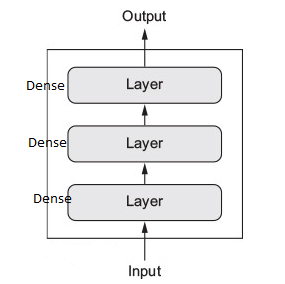Keras graph construction using functional API:

The graph contains edges and the Keras graph is also unique. The Keras graph is a directed graph in which layers act as nodes and the tensors act as edges. Defining edges is straightforward - we just need to create layer objects.

Pseudocode to specify the nodes of the graph:

from Keras.layers import Input, Dense

dense_layer_1 = Dense(units=20, activation='relu', name='dense_layer_1')

dense_layer_2 = Dense(units=20, activation='relu', name='dense_layer_2')

sigmoid_layer = Dense(units=1, activation='sigmoid', name='sigmoid_layer')

# While Input() returns a Keras tensor, Input() implicitly creates an `InputLayer` object

# which acts as a node in the Keras graph.

input_tensor = Input(shape=(10,), name='input')

Major contributors and backers for keras: